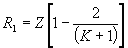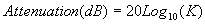# Tee Resistive Attenuator Circuits and Calculator

The formulas on this page are associated with Tee resistive attenuators. These devices can be used to reduce the amplitude of a given signal so that it can then be safely monitored on a diagnostic device (such as an oscilloscope or digitizer) without damaging the diagnostic.

These Tee resistive attenuator circuits provide a matched impedance (Z) between the source and the load (in most cases, this is 50 ohms) and the attenuation factor depends upon the proper load impedance being supplied (e.g. a 50 ohm attenuator must be terminated in 50 ohms in order to attenuate the signal to the appropriate degree). If it is left terminated in 1 Meg-ohm, the attenuation factor will not be the same as otherwise expected.

The table below shows the general equations used to determine the Tee resistive attenuator internal resistances and output voltage attenuation. As can be seen from the schematic diagram below, each attenuator consists of two equal series resistances and a different resistance shunted to ground from the node between the two series values, thus forming the “Tee” circuit.Tee Resistive Attenuator Equation for Series Resistance T-Pad Series Resistance Z is the load impedance (W) K is the attenuation factor (Vout/Vin)Tee Resistive Attenuator Equation for Shunt Resistance T-Pad Shunt Resistance All variables defined as aboveEquation for Attenuation in dB Attenuation in decibels (dB) All variables defined as above

The table below shows the R1 and R2 resistor values for common attenuator values for a 50 ohm impedance Tee resistive attenuator.

Attenuation Factor (K)R1 Series Resistance (Ohms)R2 Shunt Resistance (Ohms)
216.766.7
533.320.8
1040.910.1

For the physical implementation of these devices, precision resistors are usually selected and care must be taken in the layout to avoid stray reactance which might cause signal reflections.

The circuit schematic for the Tee resistive attenuator is shown below. In this specific model, the input waveform is a 1 MHz, 1V ac source and the values shown are for a 10X (20 dB), 50 ohm attenuator.

The results of the circuit model are shown below. V(1) is the 1V, 1 MHz, sinusoidal input voltage. The attenuator output voltages, V(RLOAD), is graphed in the second, lower plot. The waveform plot can be clicked on to open up the full size graph in a separate window.Tee Attenuator Circuit Simulation Voltage Input and Output Waveforms

The calculator below can be used to determine the proper internal resistor values for an attenuator of a given configuration (based on attenuation factor and matching impedance). It will also determine the attenuation in dB. Credit for the initial Javascript code used in the calculator is given to Ray Allen who has a number of similar useful calculators on his website, Pulsed Power Portal.

Calculate Tee Resistive Attenuator Resistance

 Parameter Value Units Inputs: z (Load Impedance) ohmskilo-ohmsmega-ohmsgiga-ohmsmilli-ohmsmicro-ohmsnano-ohms____________________ K (Attenuation Factor) Outputs: R1 (Series Resistance) ohmskilo-ohmsmega-ohmsgiga-ohmsmilli-ohmsmicro-ohmsnano-ohms____________________ R2 (Shunt Resistance) ohmskilo-ohmsmega-ohmsgiga-ohmsmilli-ohmsmicro-ohmsnano-ohms____________________ Attenuation (dB)

Output Format:

 Select Format: Scientific Engineering Fixed

Send consulting inquiries, comments, and suggestions to richard.ness@nessengr.com